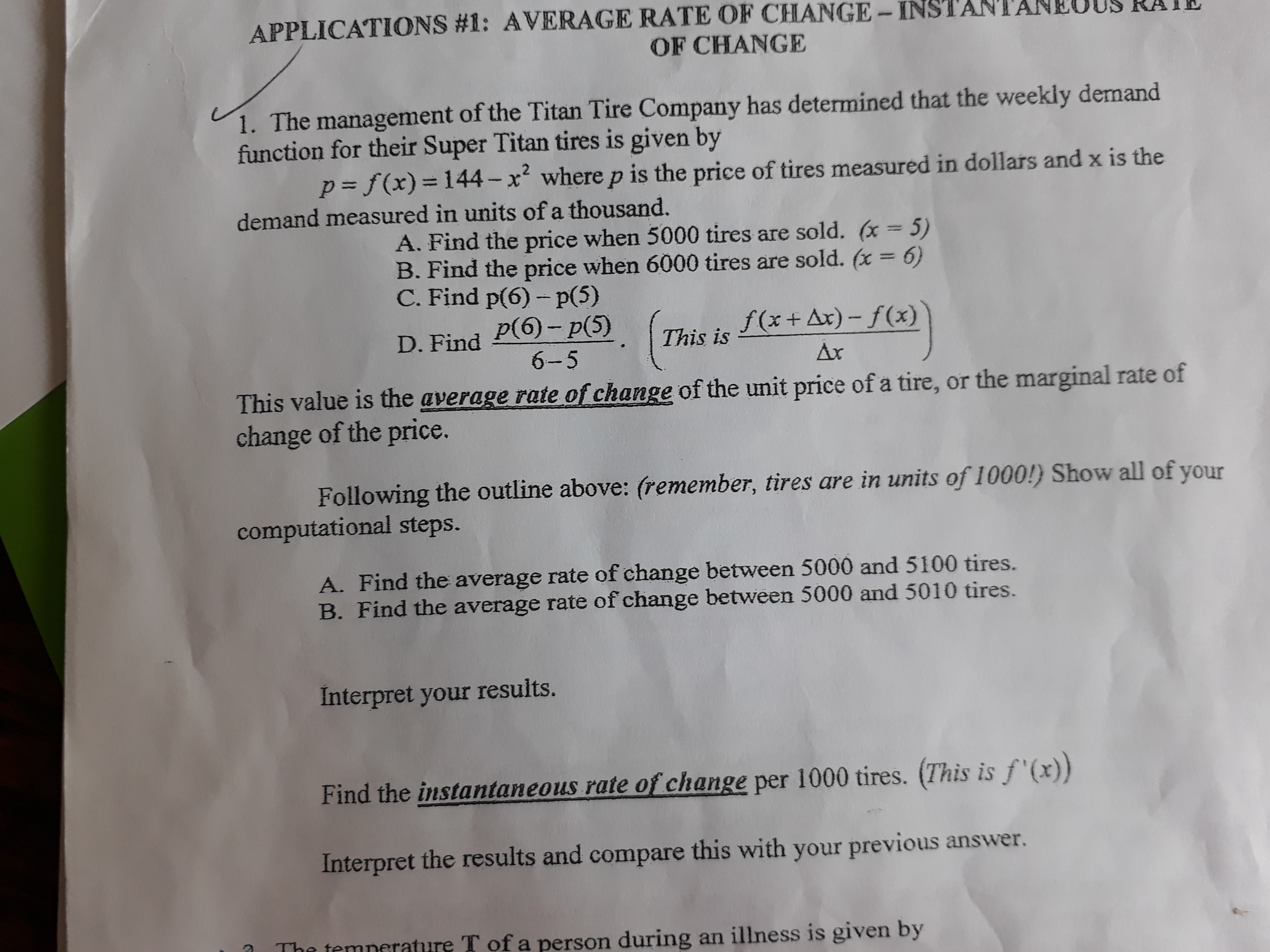# APPLICATIONS #1: AVERAGE RATE OF CHANGE- INSTANTAN OF CHANGE 1. The management of the Titan Tire Company has determined that the weekly demand function for their Super Titan tires is given by p= f(x)=144- x where p is the price of tires measured in dollars and x is the %3D %3D demand measured in units of a thousand. A. Find the price when 5000 tires are sold. (x = 5) B. Find the price when 6000 tires are sold. (x = 6) C. Find p(6) - p(5) p(6)- p(5) f(x+ Ax) - f(x) D. Find This is 6-5 Ax This value is the average rate of change of the unit price of a tire, or the marginal rate of change of the price. Following the outline above: (remember, tires are in units of 1000!) Show all of your computational steps. A. Find the average rate of change between 5000 and 5100 tires. B. Find the average rate of change between 5000 and 5010 tires. Interpret your results. Find the instantaneous rate of change per 1000 tires. (This is f '(x)) Interpret the results and compare this with your previous answer. The temnerature T of a person during an illness is given by

Questionhelp_outlineImage TranscriptioncloseAPPLICATIONS #1: AVERAGE RATE OF CHANGE- INSTANTAN OF CHANGE 1. The management of the Titan Tire Company has determined that the weekly demand function for their Super Titan tires is given by p= f(x)=144- x where p is the price of tires measured in dollars and x is the %3D %3D demand measured in units of a thousand. A. Find the price when 5000 tires are sold. (x = 5) B. Find the price when 6000 tires are sold. (x = 6) C. Find p(6) - p(5) p(6)- p(5) f(x+ Ax) - f(x) D. Find This is 6-5 Ax This value is the average rate of change of the unit price of a tire, or the marginal rate of change of the price. Following the outline above: (remember, tires are in units of 1000!) Show all of your computational steps. A. Find the average rate of change between 5000 and 5100 tires. B. Find the average rate of change between 5000 and 5010 tires. Interpret your results. Find the instantaneous rate of change per 1000 tires. (This is f '(x)) Interpret the results and compare this with your previous answer. The temnerature T of a person during an illness is given by fullscreen